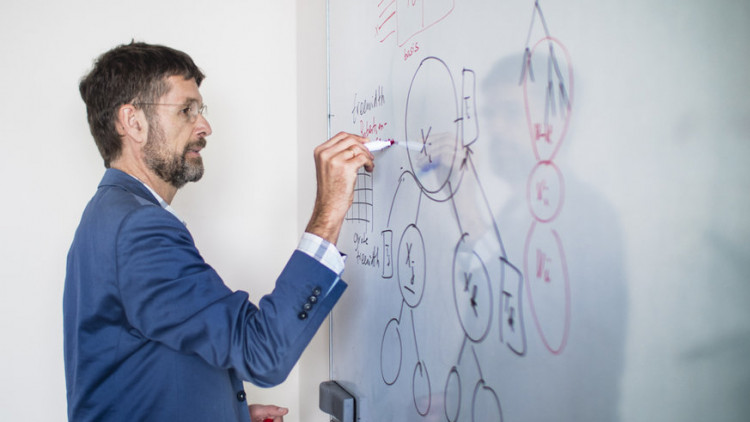# Hans L. Bodlaender

Professor Algorithms and Complexity
Department of Information and Computing Sciences
Utrecht University
the Netherlands

Foto by Ivar Pel

Research    -    Education    -    Group    -    Personal    -    Misc    -    Contact

My research focuses on Algorithms and Complexity. Many computational tasks for computers (and other computing devises) are hard and time consuming. A central question is: how can we build methods (algorithms) that solve these tasks in an efficient way? Given a specific task, we can ask: how hard is it (for a computer to solve)? Are there fast algorithms, or are all algorithms necessarily slow? How much time is needed? And, how much other resources (e.g., memory for the computation, number of parallel processes, etc.) are needed? I work and have worked on various topics in this field, including:
• Fixed parameter complexity. Suppose some parameter of our task is small: e.g., we want to solve a facility location problem (for example: find the optimal location of k hospitals on a map such that the maximum time to travel to a hospital is as small as possible; with the map and k given), but the number of facilities to be placed (k) is small. Several tasks become much easier in the case that such a parameter is small. The area of fixed parameter complexity looks at such cases and comes with faster algorithms, but also a mathematical analysis what is, or is not possible to achieve. An overview of some recent investigations into Parameterized complexity can be found here.
• Kernelization: mathematical analysis of preprocessing. A common approach when solving a hard problem is to apply preprocessing before applying computationally slow exact algorithms. The notion of kernelization makes a precise mathematical analysis of this: can we give a guarantee on the result of a `safe and sound' preprocessing method?
• Graphs and networks with a tree-like structure. Many computationally hard problems on graphs and networks appear to become significantly easier if the network (or graph) has a representation with a tree-structure. One of the most successful approaches uses the notions of tree decomposition and treewidth. My work contains algorithms to determine if such tree representations exist, and if so, find them, and look at (improved) methods to exploit these representations.
The study of the algorithmic complexity of problems, includes the design of new algorithms, the analysis of the efficiency of these algorithms, but also the use of (and design of) complexity theoretic tools to predict that algorithms with certain efficiencies can be expected not to exist.

I participate in the Center for Complex Systems Studies of Utrecht University, as member of the Advisory Board and Associated Member.

I chair the Steering Committee of the Workshop on Graph Theoretic Concepts in Computer Science, a yearly conference, since 1975, and am regular member of program committees of conferences in theoretical computer science, algorithms research, and parameterized algorithms and complexity.

You can find links to most of my papers on DBLP, or on Google Scholar. A number of early technical reports can be found here.